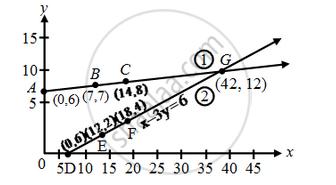# A father tells his daughter, “ Seven years ago, I was seven times as old as you were then. Also, three years from now, I shall be three times as old as you will be.” Represent this situation algebraically and graphically. - Mathematics

A father tells his daughter, “ Seven years ago, I was seven times as old as you were then. Also, three years from now, I shall be three times as old as you will be.” Represent this situation algebraically and graphically.

#### Solution

Let the present age of father be x years and that of daughter = y years

Seven years ago father’s age = (x – 7) years

Seven years ago daughter’s age = (y – 7) years

According to the problem

(x – 7) = 7(y – 7) or x – 7y = – 42 ….(1)

After 3 years father’s age = (x + 3) years

After 3 years daughter’s age = (y + 3) years

According to the condition given in the question

x + 3 = 3(y + 3) or x – 3y = 6 ….(2)

x – 7y = –42 ⇒ y=\frac { x+42 }{ 7 }

 x 0 7 14 y 6 7 8 Points A B C

x – 3y = 6   ⇒   y=\frac { x-6 }{ 3 }

 x 6 12 18 y 0 2 4 Points D E F

Plot the points A(0, 6), B(7, 7), C(14, 8) and join them to get a straight line ABC. Similarly plot the points D(6, 0), E(12, 2) and F(18,4) and join them to get a straight line DEF.Concept: Graphical Method of Solution of a Pair of Linear Equations
Is there an error in this question or solution?

#### APPEARS IN

NCERT Class 10 Maths
Chapter 3 Pair of Linear Equations in Two Variables
Exercise 3.1 | Q 1 | Page 44